Engineering ToolBox - Resources, Tools and Basic Information for Engineering and Design of Technical Applications!

# Cantilever Beams - Moments and Deflections

## Maximum reaction forces, deflections and moments - single and uniform loads.

### Cantilever Beam - Single Load at the End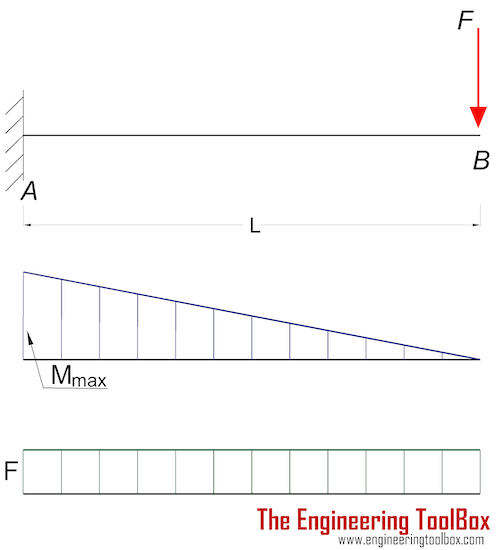#### Maximum Reaction Force

at the fixed end can be expressed as:

RA = F                              (1a)

where

RA = reaction force in A (N, lb)

F = single acting force in B (N, lb)

#### Maximum Moment

at the fixed end can be expressed as

Mmax = MA

= - F L                               (1b)

where

MA = maximum moment in A (Nm, Nmm, lb in)

L = length of beam (m, mm, in)

#### Maximum Deflection

at the end of the cantilever beam can be expressed as

δB = F L3 / (3 E I)                                      (1c)

where

δB = maximum deflection in B (m, mm, in)

E = modulus of elasticity (N/m2 (Pa), N/mm2, lb/in2 (psi))

I = moment of Inertia (m4, mm4, in4)

b = length between B and C (m, mm, in)

#### Stress

The stress in a bending beam can be expressed as

σ = y M / I                                     (1d)

where

σ = stress (Pa (N/m2), N/mm2, psi)

y = distance to point from neutral axis (m, mm, in)

M = bending moment (Nm, lb in)

I = moment of Inertia (m4, mm4, in4)

The maximum moment in a cantilever beam is at the fixed point and the maximum stress can be calculated by combining 1b and 1d to

σmax = ymax F L / I                (1e)

#### Example - Cantilever Beam with Single Load at the End, Metric Units

The maximum moment at the fixed end of a UB 305 x 127 x 42 beam steel flange cantilever beam 5000 mm long, with moment of inertia 8196 cm4 (81960000 mm4), modulus of elasticity 200 GPa (200000 N/mm2) and with a single load 3000 N at the end can be calculated as

Mmax = (3000 N)  (5000 mm)

= 1.5 107 Nmm

= 1.5 104 Nm

The maximum deflection at the free end can be calculated as

δB = (3000 N) (5000 mm)3 / (3 (2 105 N/mm2) (8.196 107 mm4))

= 7.6 mm

The height of the beam is 300 mm and the distance of the extreme point to the neutral axis is 150 mm. The maximum stress in the beam can be calculated as

σmax = (150 mm) (3000 N) (5000 mm) / (8.196 107 mm4)

= 27.4 (N/mm2)

= 27.4 106 (N/m2, Pa)

= 27.4 MPa

Maximum stress is way below the ultimate tensile strength for most steel.

### Cantilever Beam - Single Load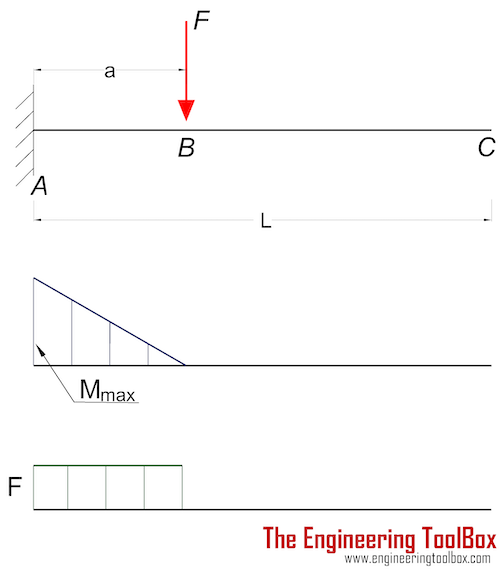#### Maximum Reaction Force

at the fixed end can be expressed as:

RA = F                              (2a)

where

RA = reaction force in A (N, lb)

F = single acting force in B (N, lb)

#### Maximum Moment

at the fixed end can be expressed as

Mmax = MA

= - F a                               (2b)

where

MA = maximum moment in A (N.m, N.mm, lb.in)

a = length between A and B (m, mm, in)

#### Maximum Deflection

at the end of the cantilever beam can be expressed as

δC = (F a3 / (3 E I)) (1 + 3 b / 2 a)                                       (2c)

where

δC = maximum deflection in C (m, mm, in)

E = modulus of elasticity (N/m2 (Pa), N/mm2, lb/in2 (psi))

I = moment of Inertia (m4, mm4, in4)

b = length between B and C (m, mm, in)

#### Maximum Deflection

at the action of the single force can be expressed as

δB = F a3 / (3 E I)                              (2d)

where

δB = maximum deflection in B (m, mm, in)

#### Maximum Stress

The maximum stress can be calculated by combining 1d and 2b to

σmax = ymax F a / I               (2e)

#### Cantilever Beam - Single Load Calculator

A generic calculator - be consistent and use metric values based on m or mm, or imperial values based on inches. Default typical values are in metric mm.

### Cantilever Beam - Uniform Distributed Load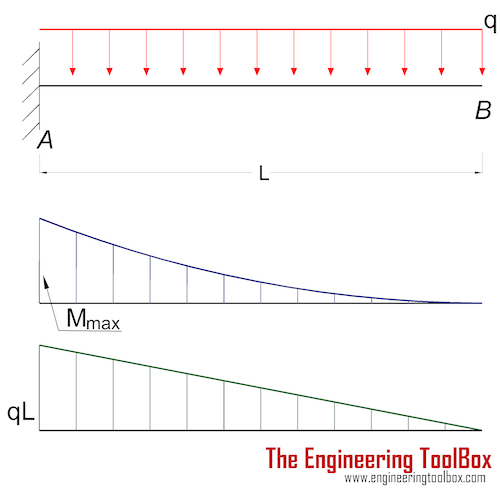#### Maximum Reaction

at the fixed end can be expressed as:

RA = q L                                (3a)

where

RA = reaction force in A (N, lb)

q = uniform distributed load (N/m, N/mm, lb/in)

L = length of cantilever beam (m, mm, in)

#### Maximum Moment

at the fixed end can be expressed as

MA = - q L2 / 2                              (3b)

#### Maximum Deflection

at the end can be expressed as

δB = q L4 / (8 E I)                               (3c)

where

δB = maximum deflection in B (m, mm, in)

#### Cantilever Beam - Uniform Load Calculator

A generic calculator - use metric values based on m or mm, or imperial values based on inches. Default typical values are in metric mm.

### More than One Point Load and/or Uniform Load acting on a Cantilever Beam

If more than one point load and/or uniform load are acting on a cantilever beam - the resulting maximum moment at the fixed end A and the resulting maximum deflection at end B can be calculated by summarizing the maximum moment in A and maximum deflection in B for each point and/or uniform load.

### Cantilever Beam - Declining Distributed Load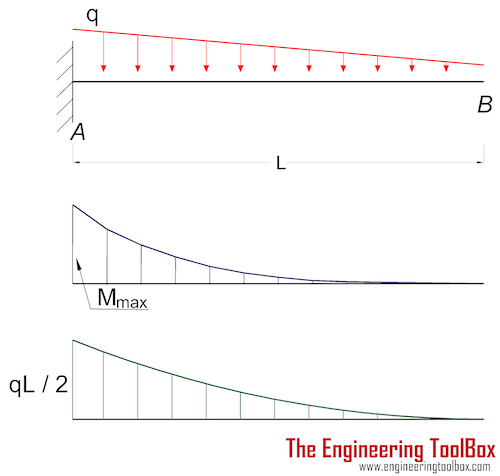#### Maximum Reaction Force

at the fixed end can be expressed as:

RA = q L / 2                              (4a)

where

RA = reaction force in A (N, lb)

q = declining distributed load - max value at A - zero at B (N/m, lb/ft)

#### Maximum Moment

at the fixed end can be expressed as

Mmax = MA

= - q L2 / 6                               (4b)

where

MA = maximum moment in A (N.m, N.mm, lb.in)

L = length of beam (m, mm, in)

#### Maximum Deflection

at the end of the cantilever beam can be expressed as

δB = q L4 / (30 E I)                                      (4c)

where

δB = maximum deflection in B (m, mm, in)

E = modulus of elasticity (N/m2 (Pa), N/mm2, lb/in2 (psi))

I = moment of Inertia (m4, mm4, in4)

### Insert beams to your Sketchup model with the Engineering ToolBox Sketchup Extension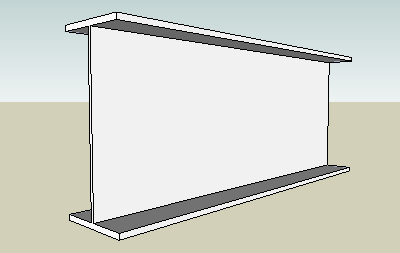## Related Topics

• ### Beams and Columns

Deflection and stress, moment of inertia, section modulus and technical information of beams and columns.

## Related Documents

• ### Area Moment of Inertia - Typical Cross Sections I

Typical cross sections and their Area Moment of Inertia.
• ### Beam Loads - Support Force Calculator

Calculate beam load and supporting forces.

• ### Center Mass

Calculate position of center mass.
• ### Continuous Beams - Moment and Reaction Support Forces

Moments and reaction support forces with distributed or point loads.
• ### Drawbridge - Force and Moment vs. Elevation

Calculate the acting forces and moments when elevating drawbridges or beams.
• ### HE-B Steel Beams

Properties of HE-B profiled steel beams.
• ### Levers

Use levers to magnify forces.
• ### Mass Moment of Inertia

The Mass Moment of Inertia vs. mass of object, it's shape and relative point of rotation - the Radius of Gyration.
• ### Square Hollow Structural Sections - HSS

Weight, cross sectional area, moments of inertia - Imperial units
• ### Steel Angles - Equal Legs

Dimensions and static parameters of steel angles with equal legs - metric units.
• ### Steel Angles - Unequal Legs

Dimensions and static parameters of steel angles with unequal legs - imperial units.
• ### Steel Angles - Unequal Legs

Dimensions and static parameters of steel angles with unequal legs - metric units.
• ### Stiffness

Stiffness is resistance to deflection.
• ### Three-Hinged Arches - Continuous and Point Loads

Support reactions and bending moments.
• ### Trusses

Common types of trusses.
• ### Weight of Beams - Stress and Strain

Stress and deformation of vertical beams due to own weight.
• ### Wood Headers - Max. Supported Weight

Weight supported by a double or triple wood headers.
• ### Young's Modulus, Tensile Strength and Yield Strength Values for some Materials

Young's Modulus (or Tensile Modulus alt. Modulus of Elasticity) and Ultimate Tensile Strength and Yield Strength for materials like steel, glass, wood and many more.

## Engineering ToolBox - SketchUp Extension - Online 3D modeling!

Add standard and customized parametric components - like flange beams, lumbers, piping, stairs and more - to your Sketchup model with the Engineering ToolBox - SketchUp Extension - enabled for use with older versions of the amazing SketchUp Make and the newer "up to date" SketchUp Pro . Add the Engineering ToolBox extension to your SketchUp Make/Pro from the Extension Warehouse !

We don't collect information from our users. More about

## Citation

• The Engineering ToolBox (2013). Cantilever Beams - Moments and Deflections. [online] Available at: https://www.engineeringtoolbox.com/cantilever-beams-d_1848.html [Accessed Day Month Year].

Modify the access date according your visit.

10.2.10